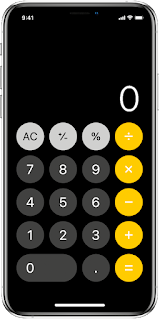## Online Calculator

CalculatorHere ,enjoy the iPhone design calculator. for the calculate not any limit . simple calculator you can use (--+) without any rules.

There is no limit to number calculation, this calculator does not work perfectly in some devices, so you can open it in browser desktop mode.

#### Click the on off button to turn the device keyboard enable or disable in the calculator below.

keyboard Enable : OFF

Simple Calculator

Addition, Subtraction, Multiplication, Divide and percentage all calculation measurement is included in our iPhone design calculator. here,you can easily calculate numbers.
If you want to learn the coding of calculator, then click on 'Learn Calculator Secure Coding' button below.

If you like this iOS Design Calculator, do let us know in the comment section below and don't forget to share this calculator tool on WhatsApp, Facebook.